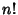## Hook Length Formula

A Formula for the number of Young Tableaux associated with a given Young Diagram. In each box, write the sum of one plus the number of boxes horizontally to the right and vertically below the box (the ``hook length''). The number of tableaux is thendivided by the product of all ``hook lengths''. The Combinatorica`NumberOfTableaux function in Mathematicaimplements the hook length formula.